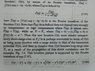# Direct correlation function

• enrikofermi
In summary, the conversation discussed the use of Fourier transforms and convolution in solving a problem related to critical phenomena. The participants also clarified the arguments of functions in the integral and how they relate to translation invariance in a homogeneous system. Overall, the conversation focused on understanding and applying the concepts of convolution and Fourier transforms in solving the problem.

#### enrikofermi

Hi all.
I'm learning something about critical phenomena and I have one problem.

I'm bad with Fourier transforms so I don't know how from 7.37 we have 7.38.
I have tryed everything I knew, but fruitless. I have attached picture of my problem.

Does anybody has any idea how I can solve this?

#### Attachments

•IMG988.jpg
67.1 KB · Views: 436
Multiply 7.37 with the denominator of the RHS and then Fourier transform using that a product in Fourier space becomes a convolution in direct space (and vice versa), i.e. the integral on the RHS of eq. 7.38.

https://en.wikipedia.org/wiki/Convolution_theorem

Thanks!
Is the Fourier transform of C (with hat) just C without hat, taking into account deffinition of C?
What make me confused are the arguments of functions in 7.38. Why r-r', and so on...?

Anybody? To help with this manybody? :)

That C and ##\hat{C}## are Fourier transforms of each other is clearly stated in the article.
r1-r2 is as valid a variable as e.g. x or y. In a homogeneous system, translation invariant quantities cannot depend on both r1 and r2 but via their difference r1-r2. I suppose this has been discussed in your text previously

Yes, I know, it was discussed earlier. But that what confused me was arguments of those functions under integral. Why are they different? And why they differ from those arguments of function outside of integral?

I think you first have to convince yourself that the integral represents a convolution.

•